﻿ 基于GPU的实时水声信道仿真实现
 舰船科学技术2017, Vol. 39Issue (12): 100-104PDF

Realization of real-time underwater acoustic channel based on GPU
BAI Jing-xian, GAO Tian-de, XIA Run-peng, LIU Lei
School of Marine Science and Technology, Northwestern Polytechnical University, Xi′an 710072, China
Abstract: The real-time performance of underwater acoustic channel estimation is very important for target tracking and positioning, underwater acoustic communication and other technologies. Firstly, this paper analyzes the propagation characteristics and models of underwater acoustic in shallow sea. Including sound velocity modeling, propagation decay modeling and the search model of the eccentric line. Secondly, this paper is based on the GPU and uses the OpenCL environment to realize the simulation. From the analysis of the results, it is proved that the modeling of the underwater eccentric line is reasonable and correct. At the same time, it fulfill the requirement of the real-time performance.
Key words: underwater acoustic channel estimation     intrinsic voice search     real-time     GPU     OpenCL
0 引　言

1 水声传播特性及信道模型分析

1.1 声速模型

 $c = 1\;450 + 4.21T - 0.037{T^2} + 1.14(S - 35) + 0.175P\text{。}$ (1)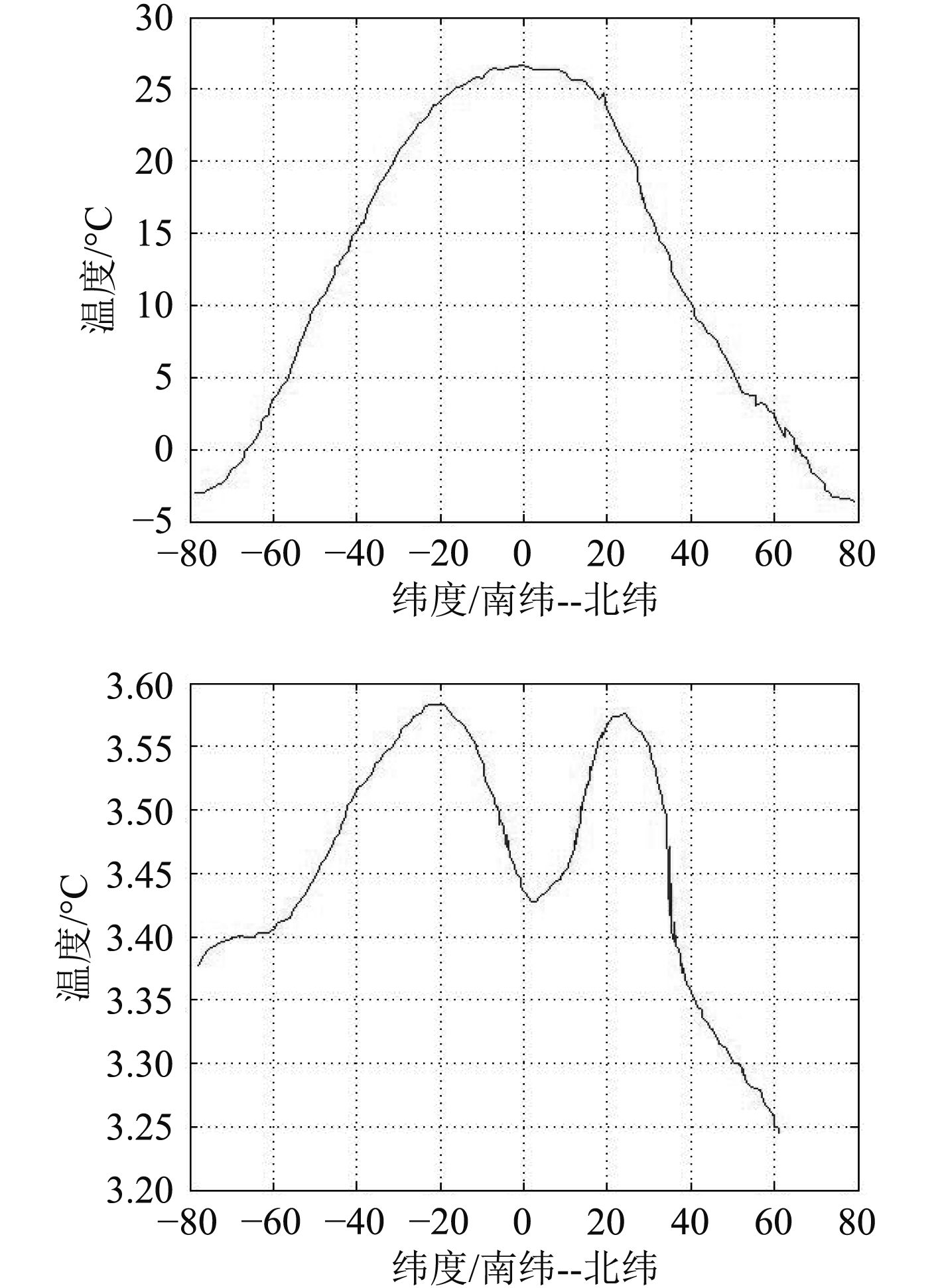图 1 海洋表面温度与盐度随纬度变化规律 Fig. 1 Variation law of ocean surface temperature and salinity with latitude
1.2 传播衰减模型

 $TL = n \cdot 101{\rm g}r\;\left( {\rm dB} \right)\text{。}$ (2)

 $a = \frac{{0.1{f^2}}}{{1 + {f^2}}} + \frac{{40{f^2}}}{{4\;100 + {f^2}}} + 2.75 \times {10^{ - 4}}{f^2} + 0.003\text{。}$ (3)

 ${V_s} = 1 - 0.45 \cdot {(f \cdot H)^{3/2}} \cdot \cos {\theta _0}\text{。}$ (4)

 - \ln \left| {{V_{b0}}(\theta )} \right| = \left\{ {\begin{aligned}& {Q \cdot \theta\text{，} \quad\quad\quad\quad\quad\quad\quad 0 < \theta < {\theta _0}}\text{，}\\& { - \ln \left| {{V_{b0}}(\theta )} \right| = {\rm{const}}\text{，}\quad{\theta _0} < \theta < \displaystyle\frac{\pi }{2}}\text{。}\end{aligned}} \right. (5)

1.3 本征声线搜索模型

 $\frac{{\cos \alpha }}{c} = \frac{{\cos {\alpha _0}}}{{{c_0}}} = const \text{。}$ (6)

 ${\rm tan}\alpha = \sqrt {({n^2}(z) - {{\cos }^2}{\alpha _1}/\cos {\alpha _1}}\text{，}$ (7)

 $x = \cos {\alpha _1}\int_{{z_1}}^z {\frac{{{\rm d}z}}{{\sqrt {({n^2}(z) - {{\cos }^2}{\alpha _1}} }}} \text{。}$ (8)

 $t = \int {\frac{{{\rm d}s}}{c}} = \int_{{z_1}}^z {\frac{{{\rm d}z}}{{c(z)\sin \alpha (z)}}}\text{，}$ (9)
 $S = \int {{\rm d}s} = \int_{{z_1}}^z {\frac{{{\rm d}z}}{{\sin \alpha (z)}}}\text{。}$ (10)

 $t = \frac{1}{{{c_1}}}\int_{{z_1}}^z {\frac{{{n^2}(z){\rm d}z}}{{\sqrt {({n^2}(z) - {{\cos }^2}{\alpha _1}} }}}\text{。}$ (11)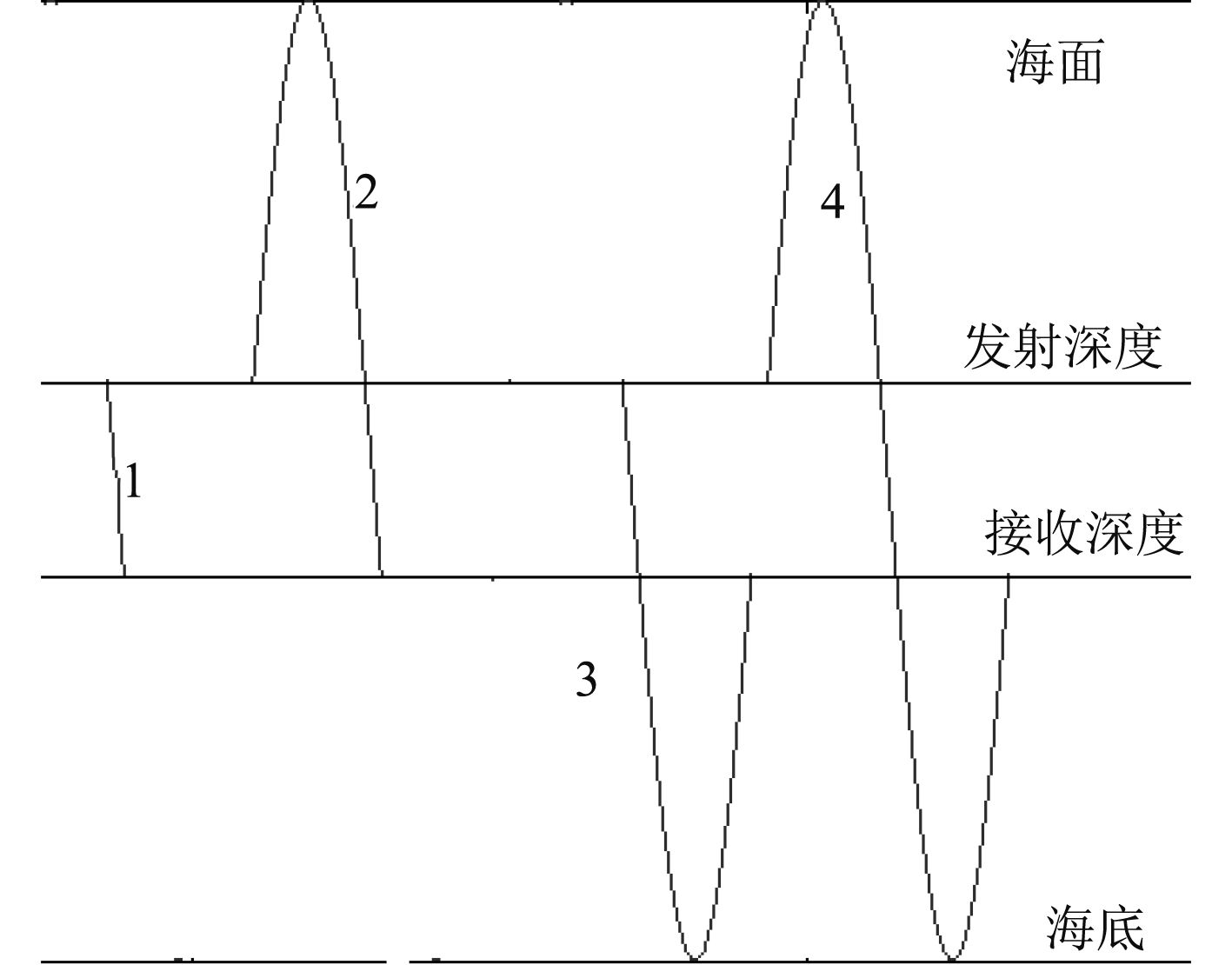图 2 本征声线的几种到达形式 Fig. 2 Several forms of the sign of the eigen ray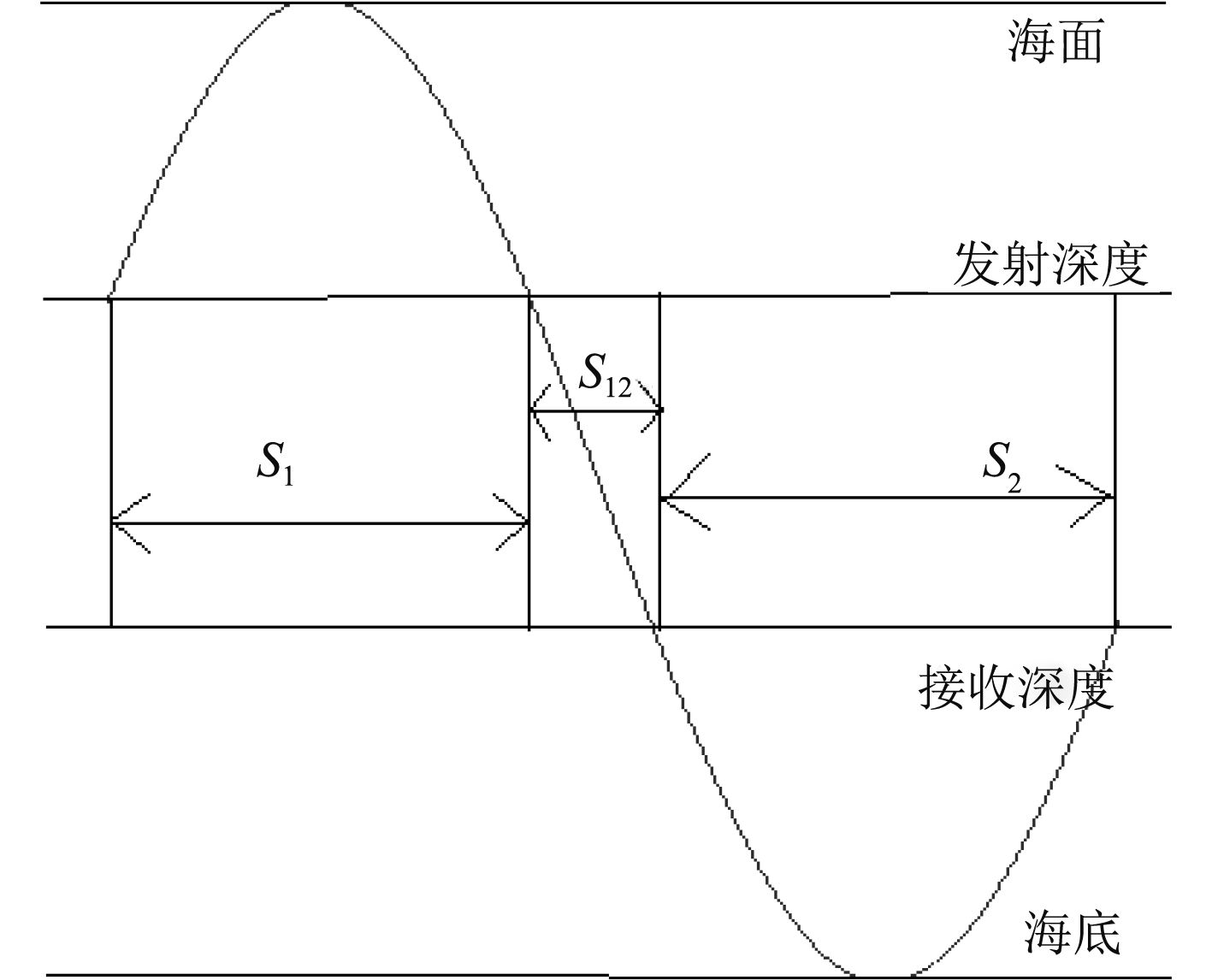图 3 4种子跨度的具体形式 Fig. 3 Four specific forms of subspecies

 $D = mS + a{S_1} + {S_{12}} + b{S_2}\text{。}$ (12)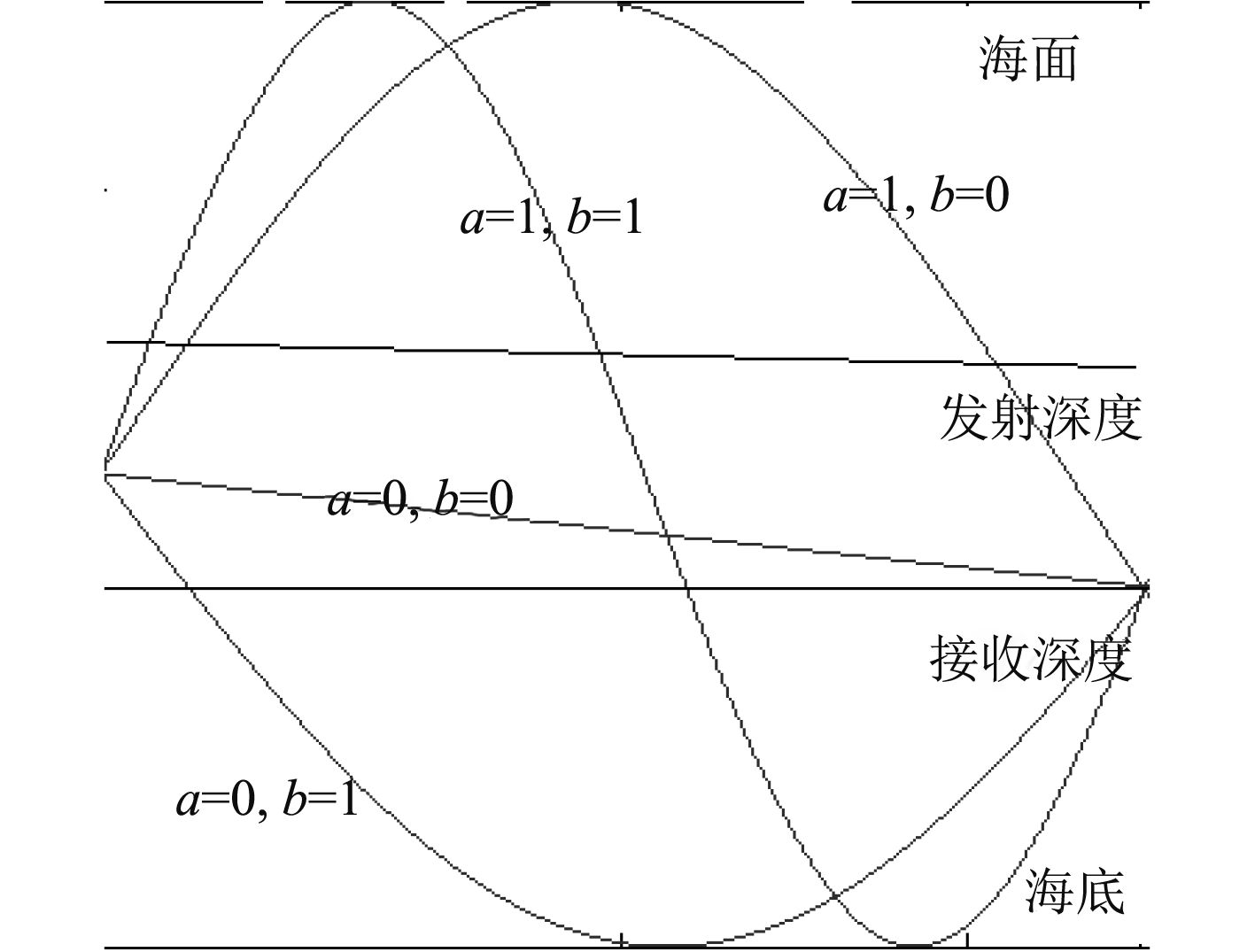图 4 几种声线到达形式声线轨迹 Fig. 4 Several sound lines arrive in the form of sound line trajectory

2 水声信道建模仿真实验 2.1 仿真实验条件

2.2 仿真实验过程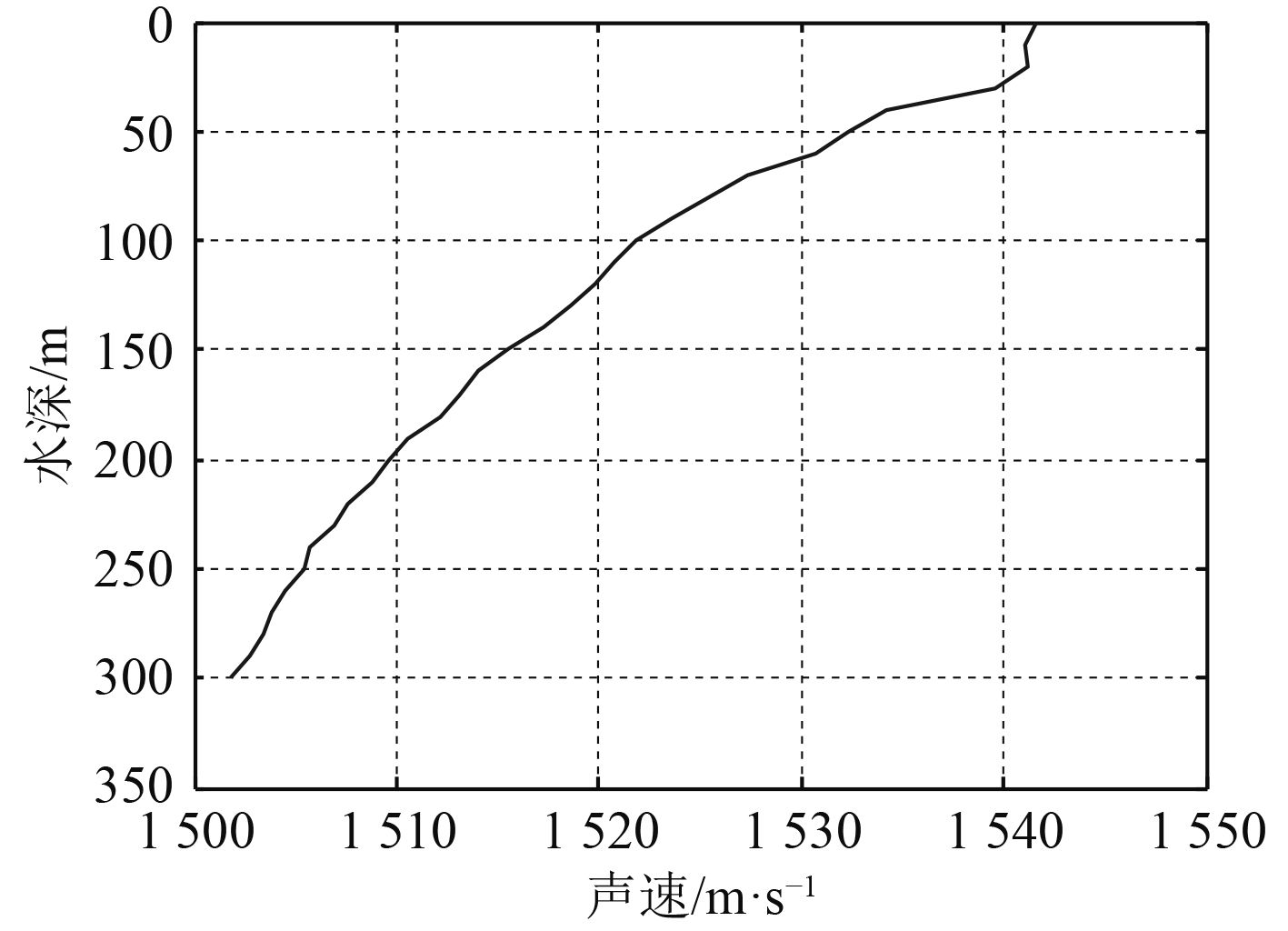图 5 水下声速梯度 Fig. 5 Underwater velocity gradient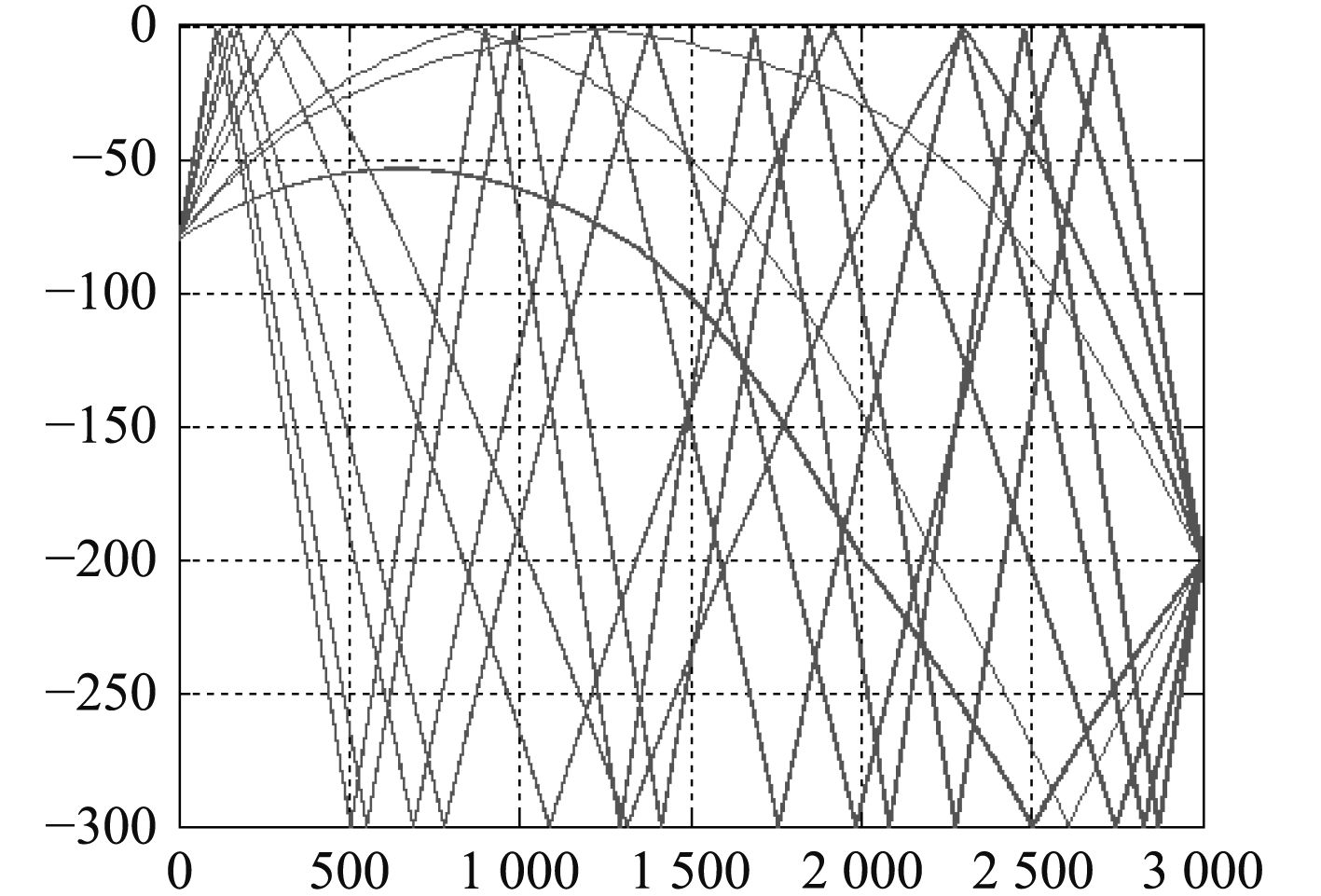图 6 声源-目标传播本征声线 Fig. 6 Sound source-target propagation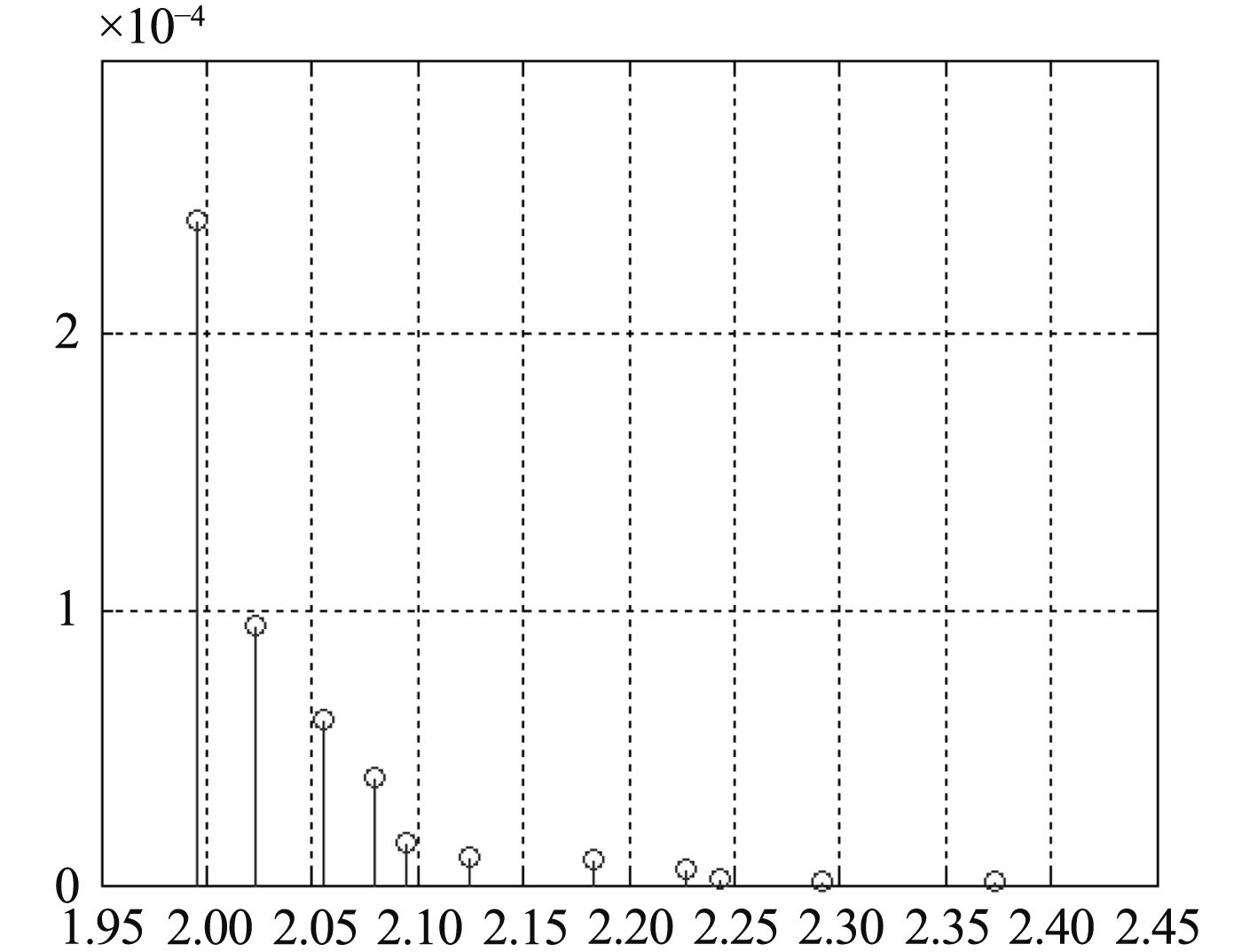图 7 声源-目标信道冲激响应 Fig. 7 Sound source-target channel impulse response
2.3 仿真实验结果分析表 1 声源-目标信道时延-衰减结果 Tab.1 Sound source-target channel delay-attenuation result

3 水声信道估计实时性实现 3.1 OpenCL简介

3.2 结果分析表 2 不同平台上信道模拟用时对比 Tab.2 Time comparison of channel simulation on different platforms

4 结　语

  兰英, 章新华, 熊鑫. 浅海水声多途信道建模与仿真[J]. 《舰船科学技术》, 2010, 32 (9): 120–122. LAN Ying, ZHANG Xin-hua, XIONG Xin. Modeling and simulation on shallow water acoustic multi-path channels[J]. 《Editorial Department of Ship Science and Technology》, 2010, 32 (9): 120–122.  蒋德军, 胡涛. 时延估计技术及其在多途环境中的应用[J]. 声学学报, 2001, 26 (1): 34–40. JIANG De-jun, HU Tao. Time-delay estimation and its application in multipath environment[J]. Journal of Acoustics, 2001, 26 (1): 34–40.  CHEN J T, PAULRAJ A, REDDY U. Multichannel maximum-likelihood sequence estimation(MLSE) equalizer for GSM using a parametric channel model[J]. Communications, IEEE Transactions on, 1999, 47 (1): 53–63. DOI: 10.1109/26.747813  CHAMPAGNE B, EIZENMAN E, PASUPATHY S. Exact maximum likelihood time delay estimation for short observation intervals[J]. Signal Processing, IEEE Transactions on, 1991, 39 (6): 1245–1257. DOI: 10.1109/78.136531  MANICKAM T G, VACCARO R J, TUFTS D W. A least-squares algorithm for multipath time-delayestimation[J]. Signal Processing, IEEE Transactions on, 1994, 42 (11): 3229–3233. DOI: 10.1109/78.330381  WU R, LI J. Time-delay estimation via optimizing highly oscillatory cost functions[J]. Oceanic Engineering, IEEE Journal of, 1998, 23 (3): 235–244. DOI: 10.1109/48.701196  EVANS A, FISCHL R. Optimal least squares time-domain synthesis of recursive digital filters[J]. Audio and Electroacoustics, IEEE Transactions on, 1973, 21 (1): 61–65. DOI: 10.1109/TAU.1973.1162433  SCHMIDT R O. Multiple emitter location and signal parameter estimation[J]. Antennas and Propagation, IEEE Transactions on, 1986, 34 (3): 276–280. DOI: 10.1109/TAP.1986.1143830  FEDER M, WEINSTEIN E. Parameter estimation of superimposed signals using the EM algorithm[J]. Acoustics, Speech and Signal Processing, IEEE Transactions on, 1988, 36 (4): 477–489. DOI: 10.1109/29.1552  SENMOTO S, CHILDERS D G. Signal resolution via digital inverse filtering[J]. Aerospace and Electronic Systems, IEEE Transactions on, 1972 (5): 633–640.  林旺生. 水声信道仿真与声线修正技术研究[D]. 哈尔滨: 哈尔滨工程大学, 2009.  欧晓丽. 水声信道建模及其仿真平台的实现[D]. 厦门: 厦门大学, 2007.  王百合, 冯西安, 黄建国, 孙静, 等. 一种分层海洋中求取本征声线的新方法[J]. 微处理机, 2006 (1): 63–65. WANG Bai-he, FENG Xi-an, HUANG Jianguo, SUN Jing, et al. A new method for finding the intrinsic line of sound in a layered ocean[J]. Microprocessor, 2006 (1): 63–65.  魏莉, 许芳, 孙海信. 水声信道的研究与仿真[J]. 声学技术, 2008 (01): 25–29. WEI Li, XU Fang, SUN Haixin. Research and simulation of underwater acoustic channel[J]. Acoustics, 2008 (01): 25–29.  陈钢, 吴百锋. 面向OpenCL模型的GPU性能优化[J]. 《计算机辅助设计与图形学学报》, 2011, 23 (4): 571–581. CHEN Gang, WU Baifeng. GPU performance optimization for OpenCL model[J]. Journal of Computer Aided Design and Graphics, 2011, 23 (4): 571–581.